Next: Radiation and Scattering Up: Wave Propagation in Inhomogeneous Previous: Jeffries Connection Formula

# Exercises

1. Consider an electromagnetic wave propagating through a nonuniform dielectric medium whose dielectric constant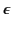is a function of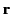. Demonstrate that the associated wave equations take the form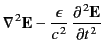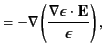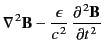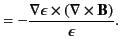2. Suppose that a light-ray is incident on the front (air/glass) interface of a uniform pane of glass of refractive index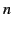at the Brewster angle. Demonstrate that the refracted ray is also incident on the rear (glass/air) interface of the pane at the Brewster angle.

3. Consider an electromagnetic wave obliquely incident on a plane boundary between two transparent magnetic media of permeabilities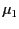and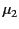. Find the coefficients of reflection and transmission as functions of the angle of incidence for the wave polarizations in which all electric fields are parallel to the boundary and all magnetic fields are parallel to the boundary. Is there a Brewster angle? If so, what is it? Is it possible to obtain total reflection? If so, what is the critical angle of incidence required to obtain total reflection?

4. A medium is such that the product of the phase and group velocities of electromagnetic waves is equal to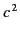at all wave frequencies. Demonstrate that the dispersion relation for electromagnetic waves takes the formwhere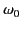is a constant.

5. Demonstrate that if the equivalent height of reflection in the ionosphere varies with the angular frequency of the wave as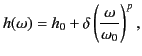where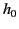,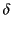, andare positive constants, then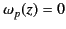for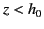, and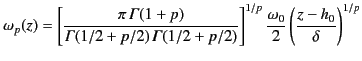for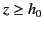. Here,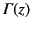is a Gamma function.

6. Suppose that the refractive index,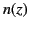, of the ionosphere is given by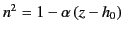for, and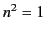for, where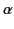andare positive constants, and the Earth's magnetic field and curvature are both neglected. Here,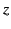measures altitude above the Earth's surface.
1. A point transmitter sends up a wave packet at an angle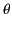to the vertical. Show that the packet returns to Earth a distance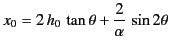from the transmitter. Demonstrate that if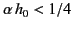then for some values of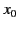the previous equation is satisfied by three different values of. In other words, wave packets can travel from the transmitter to the receiver via one of three different paths. Show that the critical case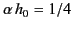corresponds to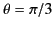and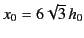.
2. A point radio transmitter emits a pulse of radio waves uniformly in all directions. Show that the pulse first returns to the Earth a distance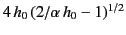from the transmitter, provided that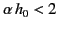.Next: Radiation and Scattering Up: Wave Propagation in Inhomogeneous Previous: Jeffries Connection Formula
Richard Fitzpatrick 2014-06-27